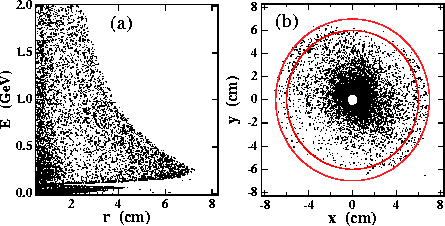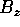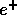Next: Results Up: Simulation by ABEL Previous: Beam-beam effect

## Tracking in a solenoidal magnetic field

In order to estimate the actual sensitivity, we must take into account the helical trajectory in the solenoidal magnet of the detector. We assume a uniform field of 2 Tesla parallel to the z-axis. We detect the particle positions 1m downstream from the IP,i.e.  z=+1m.Figure 5:   Distributions of scattered particles at z=+1m, where particles with r > 0.5cm are plotted. The particles are tracked in helical trajectories in a magnetic field of=2 tesla. (a) is for a scatter plot on the plane of the energy(E) and the radial distance(r), and (b) is for a plot on the plane of x and y, where two concentric circles of 6 and 7cm radii are also shown just for an eye guide.

Figures 5(a) and (b) show the radial and azimuthal angular distributions of the particles, respectively. There is a clear maximum radial boundary as a function of the energy in Fig.5(a), as expected from the Eq.14. Outside of the boundary only a relatively small number of the particles can be seen, which have inherent angles larger than the beam-beam deflection angle. We can also see another dense region at r<1 cm corresponding to the opposite-charge particles(), as described in the previous section. The most interesting particles are distributed at the maximum radial distance of around r=7cm. Since they have almost the same magnitude as the energy, as clearly shown in Fig.5(a), their original azimuthal distribution should be preserved even after they flowed in the magnetic field. Actually, this expectation is realized as in Fig.5(b), where we can clearly see two sides of the depleted region, especially for 6<r<7cm. The two sides are sitting on a line rotated counter-clockwise by70, which corresponds to the horizontal direction.Next: Results Up: Simulation by ABEL Previous: Beam-beam effect

Toshiaki Tauchi
Sat Dec 21 00:34:16 JST 1996